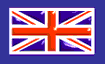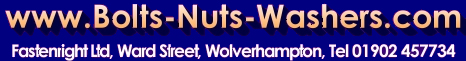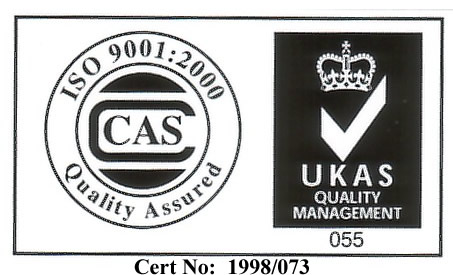[Home]   [About Us]   [Catalogue Request]  [Enquiry form]   [Contact Us]   [ISO]   [Export Department]
 Parallel Keys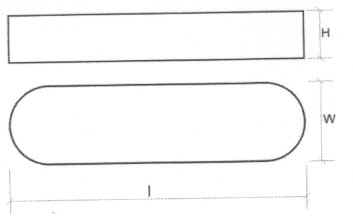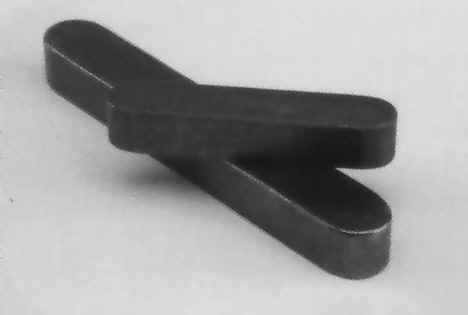Fastenright Limited Your Uk Source For Fixings & Fasteners.
 Technical Data & Available Sizes Stock range is to DIN 6885A Square ended keys or keys with   one rounded end and one square end are available.  Detailed Below Round Ended                                     DIN6885A Square Ended                                    DIN6885B One Round, One Square End           DIN 6885AB

SIZES AVAILABLE

 WIDTH 2 3 4 5 6 8 10 12 14 16 18 20 22 25 28 32 36 40 45 HEIGHT 2 3 4 5 6 7 8 8 9 10 11 12 14 14 16 18 20 22 25 LENGTH 6 x x x 8 x x x 10 x x x x x x 12 x x x x x x 14 x x x x x x x 15 x x x x x x x 16 x x x x x x x 18 x x x x x x x 20 x x x x x x x x 22 x x x x x x x x 25 x x x x x x x x x 28 x x x x x x x x x 30 x x x x x x x x x x 32 x x x x x x x x x x 35 x x x x x x x x x x 40 x x x x x x x x 45 x x x x x x x x x x 50 x x x x x x x x x x 55 x x x x x x x x x 60 x x x x x x x x x x x 65 x x x x x x x x x x x x 70 x x x x x x x x x x x x 75 x x x x x x x x x x x 80 x x x x x x x x x x 85 x x x x x x x x x x 90 x x x x x x x x x x 95 x x x x x x x x x x x 100 x x x x x x x x x x x 110 x x x x x x x x x x x x x 120 x x x x x x x x x x x x x 130 x x x x x x x x x x x x x 140 x x x x x x x x x x x x x 150 x x x x x x x x x x x x x 160 x x x x x x x x x x x x x 170 x x x x x x x x x x x x x 180 x x x x x x x x x x x x x